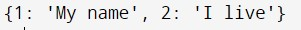# Python字典的交集

## Python字典

• 键应该是一个单一的元素。
• 值可以是任何类型，如列表、整数、元组等。

``````dict = {'Name': 'John', 'Age': 18, 'Grade': '13'}
``````

## 在 Python 中使用字典理解法来交叉两个字典

• ##### 接下来，将`dict01` 的键视为`k1` 并执行`for` 循环，检查`dict01` 中的`k1` 是否存在于`dict02` 中。如果它确实有共同的键，它的值将被推入一个新的字典，称为`intersection` (这个字典可以根据需要选择)。

``````# inititializing the dictionaries
dict01 = {'A': 1, 'B': 6, 'C': 3, 'D': 5}
dict02 = {'A': 1, 'C': 4, 'D': 5, 'E': 2}
# printing original dictionary
print ("First original dictionary: ", dict01)
print ("Second original dictionary: ", dict02)
# performing intersection
intersection = {k1:dict01[k1] for k1 in dict01
if k1 in dict02}
# printing output
print ("Intersected Dictionary: ", str(intersection))
``````

``````# inititializing the dictionaries
dict01 = {1:'My', 2:'I', 3:'age'}
dict02 = {1:'name', 2:'live', 4:'year'}
#defining a function to merge strings
def mergeStrings(str01,str02):
return str01+ ' '+ str02
# performing intersection
intersectString = {k1:mergeStrings(dict01[k1],dict02[k1]) for k1 in dict01.keys() if k1 in dict02}
# printing output
print(intersectString)
``````## 在Python中使用Bitwise`&` 操作符来交叉两个字典

• ##### 最后，打印新的字典。

``````# inititializing dictionary
dict01 =  {'A': 1, 'B': 6, 'C': 3, 'D': 5}
dict02 =  {'A': 1, 'C': 4, 'D': 5, 'E': 2}
# printing original dictionary
print ("First original dictionary: ", dict01)
print ("Second original dictionary: ", dict02)
# performing intersection
intersection = dict(dict01.items() & dict02.items())
# printing output
print ("Intersected Dictionary", str(intersection))
``````

## 在Python中使用Set`intersection()` 方法来相交两个字典

set`intersection()` 方法返回一个集合，该集合由同时存在于集合`m``n` 中的元素组成，如下所示：

``````m = {'A': 1, 'B': 6, 'C': 3, 'D': 5}
n = {'A': 1, 'D': 5, 'E': 2}
setM = set( m )
setN = set( n )
setM.intersection( setN )
for item in setM.intersection(setN):
print(item)
``````

## 在Python中用于交叉多个字典的方法

``````# inititializing dictionary
dict01 =  {'A': 1, 'B': 6, 'C': 3, 'D': 5}
dict02 =  {'A': 1, 'C': 4, 'D': 5, 'E': 2}
dict03 =  {'A': 1, 'C': 9, 'D': 5, 'E': 1}
# printing original dictionary
print ("First original dictionary: ", dict01)
print ("Second original dictionary: ", dict02)
print ("Third original dictionary: ", dict03)
# performing intersection
intersection = dict(dict01.items() & dict02.items() & dict03.items())
# printing output
print ("Intersected Dictionary", str(intersection))
``````

``````m = {'A': 1, 'B': 6,'D': 5, 'E': 2}
n = {'A': 1, 'D': 5, 'E': 2}
t = {'A': 1,'C': 4, 'D': 5, 'E': 2}
setM = set( m )
setN = set( n )
setT = set( t )
setM.intersection( setN ).intersection( setT)
for item in setM.intersection( setN ).intersection( setT):
print(item)
``````